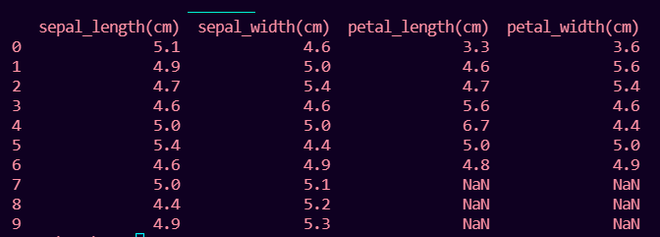# How to Fix: Length of values does not match length of index

• Last Updated : 22 Nov, 2021

In this article we will fix the error: The length of values does not match the length of the index in Python.

## Python3

 `# importing pandas ` `import` `pandas as pd ` ` `  `sepal_length ``=` `[``5.1``, ``4.9``, ``4.7``, ``4.6``, ``5.0``, ``5.4``,  ` `                ``4.6``, ``5.0``, ``4.4``, ``4.9``] ` `sepal_width ``=` `[``4.6``, ``5.0``, ``5.4``, ``4.6``, ``5.0``, ``4.4``,  ` `               ``4.9``, ``5.1``, ``5.2``, ``5.3``] ` `petal_length ``=` `[``3.3``, ``4.6``, ``4.7``, ``5.6``, ``6.7``, ``5.0``, ``4.8``] ` `petal_width ``=` `[``3.6``, ``5.6``, ``5.4``, ``4.6``, ``4.4``, ``5.0``, ``4.9``] ` ` `  `# DataFrame with 2 columns ` `df ``=` `pd.DataFrame({``'sepal_length(cm)'``: sepal_length, ` `                   ``'sepal_width(cm)'``: sepal_width}) ` ` `  `df[``'petal_length(cm)'``] ``=` `petal_length ` `df[``'petal_width(cm)'``] ``=` `petal_width ` ` `  `print``(df) `

Output:

`ValueError: Length of values (7) does not match length of index (10)`

## Reason for the error :

The length of the index of the pandas DataFrame(i.e length of the column of present DataFrame) which is 10 in this case is not equal to the length of the new list or NumPy array which is 7 in this case.

`pd.Index.size!=len(petal_width)`

## Fixing the error:

This error can be fixed by preprocessing the new list or NumPy array that is going to be a column of the DataFrame by using the pandas Series() function which actually converts the list or NumPy array into the size of the DataFrame column length by adding NaN if list or NumPy array has lesser length else if the list or NumPy has greater length then it takes the list or NumPy array with the length of columns of the pandas dataframe.

Syntax For list:

Consider list1 as a python list

`df['new_column'] = pd.Series(list1)`

Syntax For NumPy array:

Consider numarr as a numpy array

`df['new_column'] = pd.Series(numarr)`

## Python3

 `# importing pandas ` `import` `pandas as pd ` ` `  `# importing numpy ` `import` `numpy as np ` `sepal_length ``=` `[``5.1``, ``4.9``, ``4.7``, ``4.6``, ``5.0``, ``5.4``, ` `                ``4.6``, ``5.0``, ``4.4``, ``4.9``] ` `sepal_width ``=` `[``4.6``, ``5.0``, ``5.4``, ``4.6``, ``5.0``, ``4.4``, ` `               ``4.9``, ``5.1``, ``5.2``, ``5.3``] ` `petal_length ``=` `[``3.3``, ``4.6``, ``4.7``, ``5.6``, ``6.7``, ``5.0``, ``4.8``] ` ` `  `# numpy array of length 7 ` `petal_width ``=` `np.array([``3.6``, ``5.6``, ``5.4``, ``4.6``, ``4.4``,  ` `                        ``5.0``, ``4.9``]) ` ` `  `# DataFrame with 2 columns of length 10 ` `df ``=` `pd.DataFrame({``'sepal_length(cm)'``: sepal_length, ` `                   ``'sepal_width(cm)'``: sepal_width}) ` ` `  `# Adding list to pandas DataFrame ` `df[``'petal_length(cm)'``] ``=` `pd.Series(petal_length) ` ` `  `# Adding numpy array to pandas DataFrame ` `df[``'petal_width(cm)'``] ``=` `pd.Series(petal_width) ` ` `  `print``(df) `

Output:My Personal Notes arrow_drop_up
Recommended Articles
Page :